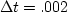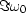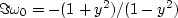Next: PRECISION EXHAUSTION Up: INTRODUCTION TO ALL-PASS FILTERS Previous: INTRODUCTION TO ALL-PASS FILTERS

## Notch filter

A notch filter''

rejects a narrow frequency band and leaves the rest of the spectrum little changed. The most common example is 60-Hz noise from power lines. Another is low-frequency ground roll. Such filters can easily be made using a slight variation on the all-pass filter. In the all-pass filter, the pole and zero have equal (logarithmic) relative distances from the unit circle. All we need to do is put the zero closer to the circle. Indeed, there is no reason why we should not put the zero right on the circle: then the frequency at which the zero is located is exactly canceled from the spectrum of input data.

Narrow-band filters and sharp cutoff filters should be used with caution. An ever-present penalty for using such filters is that they do not decay rapidly in time. Although this may not present problems in some applications, it will certainly do so in others. Obviously, if the data-collection duration is shorter than or comparable to the impulse response of the narrow-band filter, then the transient effects of starting up the experiment will not have time to die out. Likewise, the notch should not be too narrow in a 60-Hz rejection filter. Even a bandpass filter (an example of which, a Butterworth filter, is implemented in chapter) has a certain decay rate in the time domain which may be too slow for some experiments. In radar and in reflection seismology, the importance of a signal is not related to its strength. Late arriving echoes may be very weak, but they contain information not found in earlier echoes. If too sharp a frequency characteristic is used, then filter resonance from early strong arrivals may not have decayed enough by the time the weak late echoes arrive.

A curious thing about narrow-band reject filters is that when we look at their impulse responses, we always see the frequency being rejected! For example, look at Figure 15. The filter consists of a large spike (which contains all frequencies) and then a sinusoidal tail of polarity opposite to that of the frequency being rejected.notch2
Figure 15
Top: a zero on the real frequency axis and a pole just above it give a notch filter; i.e., the zeroed frequency is rejected while other frequencies are little changed. Bottom: the notch has been broadened by moving the pole further away from the zero. (This notch is at 60 Hz, assumings.)

The vertical axis in the complex frequency plane in Figure 15 is not exactly.  Instead it is something like the logarithm of.The logarithm is not precisely appropriate either because zeros may be exactly on the unit circle. I could not devise an ideal theory for scaling,so after some experimentation, I chose,where y is the vertical position in a window of vertical range 0<y<1. Because of the minus sign, the outside of the unit circle is above theaxis, and the inside of the unit circle is below it.

## EXERCISES:

1. Find a three-term real feedback filter to reject 59-61 Hz on data that is sampled at 500 points/s. (Try for about 50% rejection at 59 and 61.) Where are the poles? What is the decay time of the filter?Next: PRECISION EXHAUSTION Up: INTRODUCTION TO ALL-PASS FILTERS Previous: INTRODUCTION TO ALL-PASS FILTERS
Stanford Exploration Project
10/21/1998